Constructing a triangle when 2 sides and angle between is known (SAS)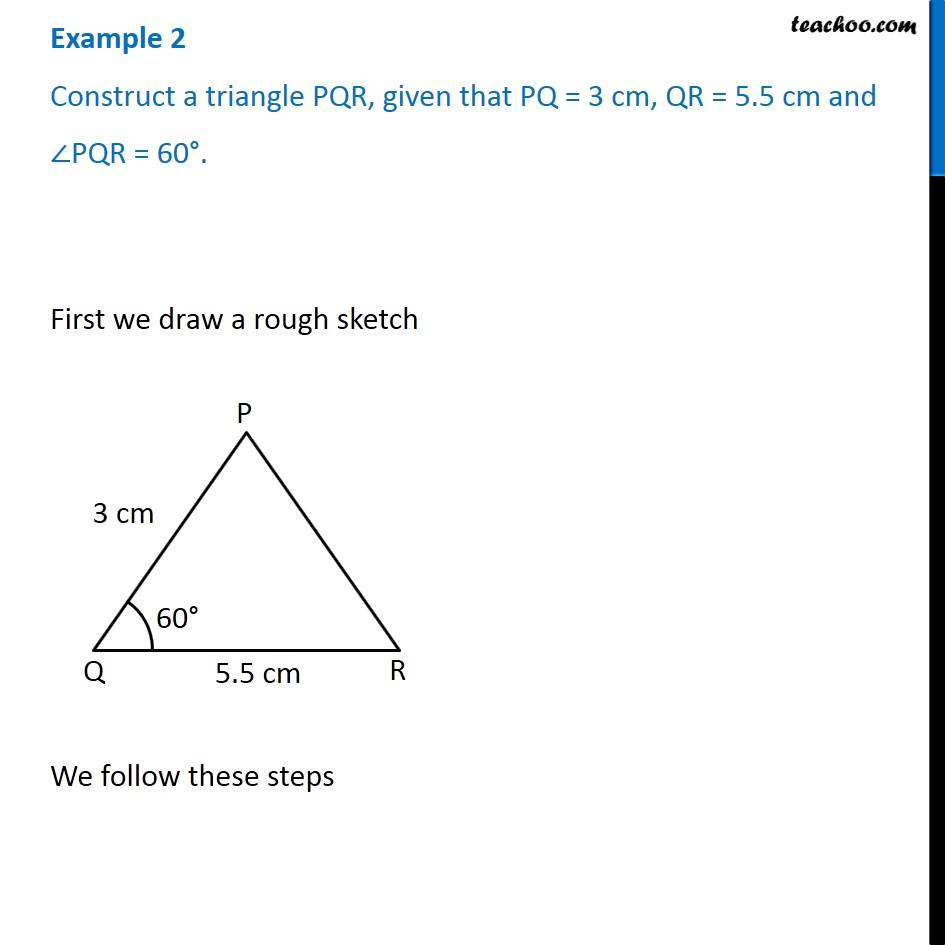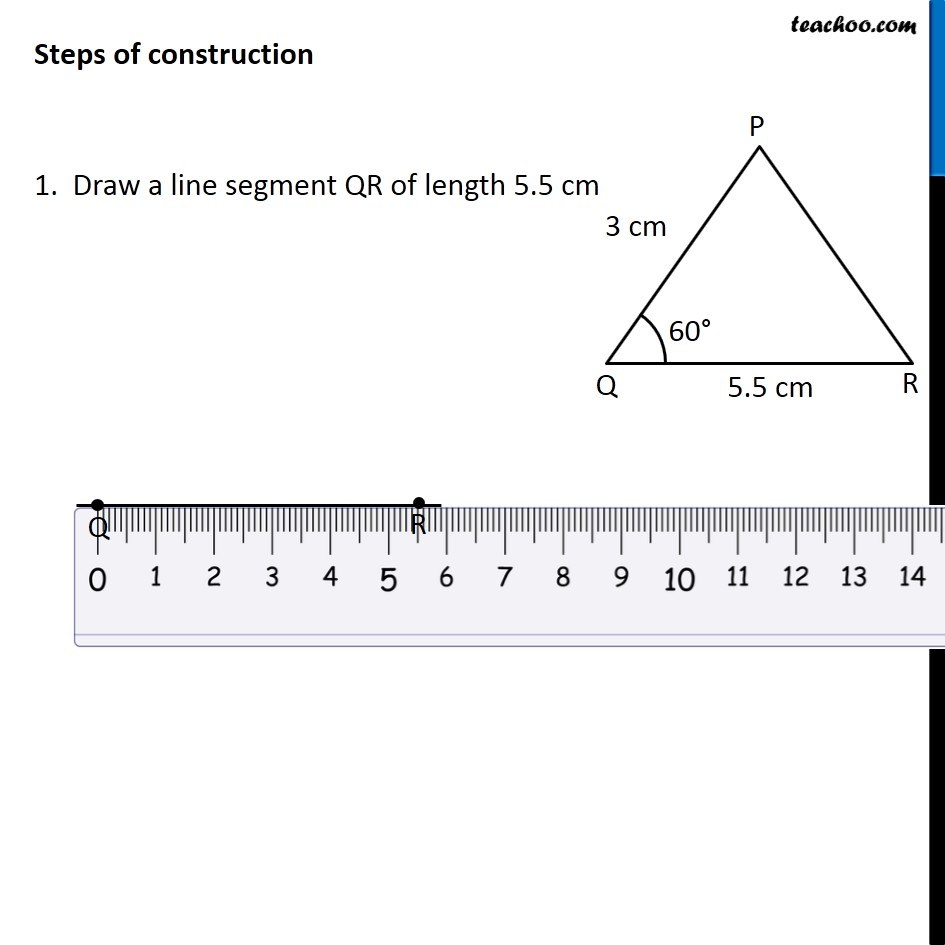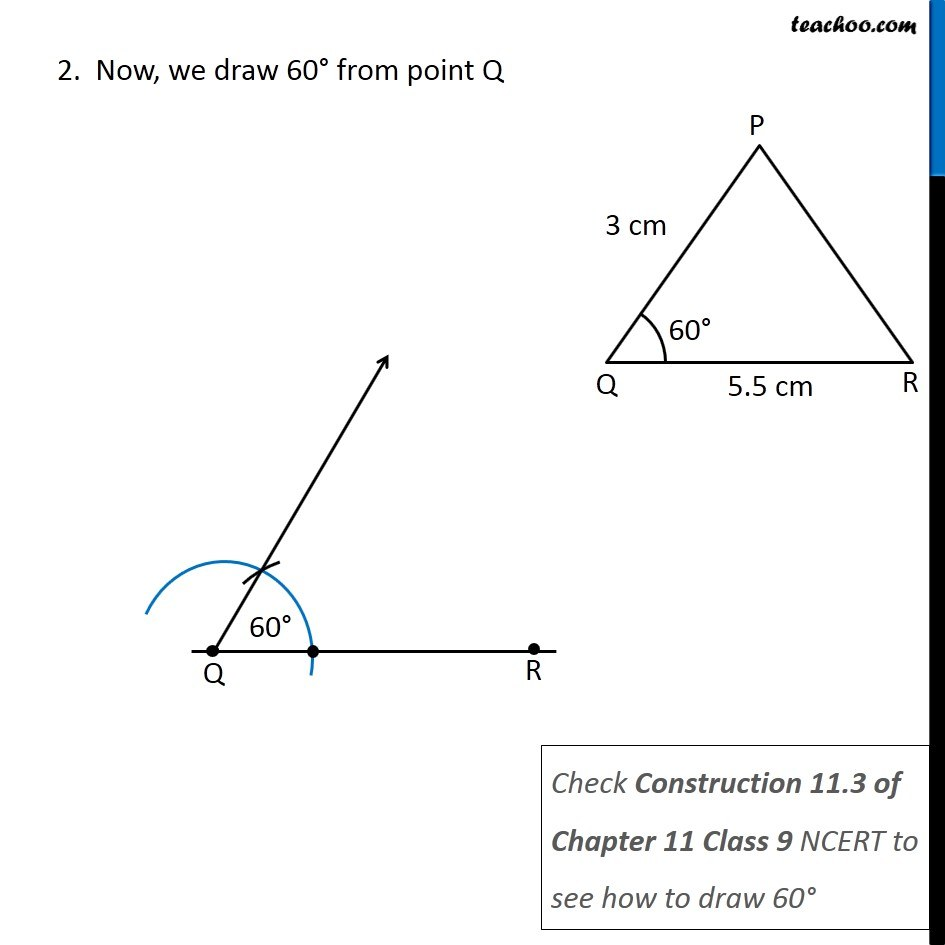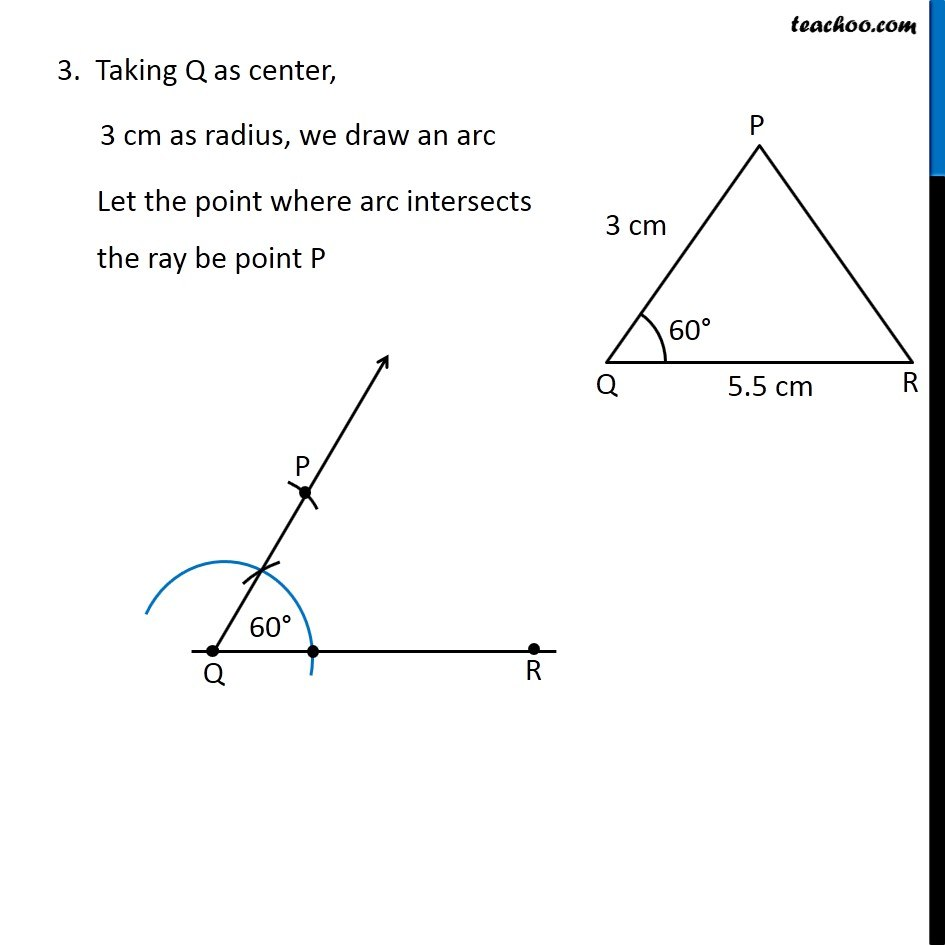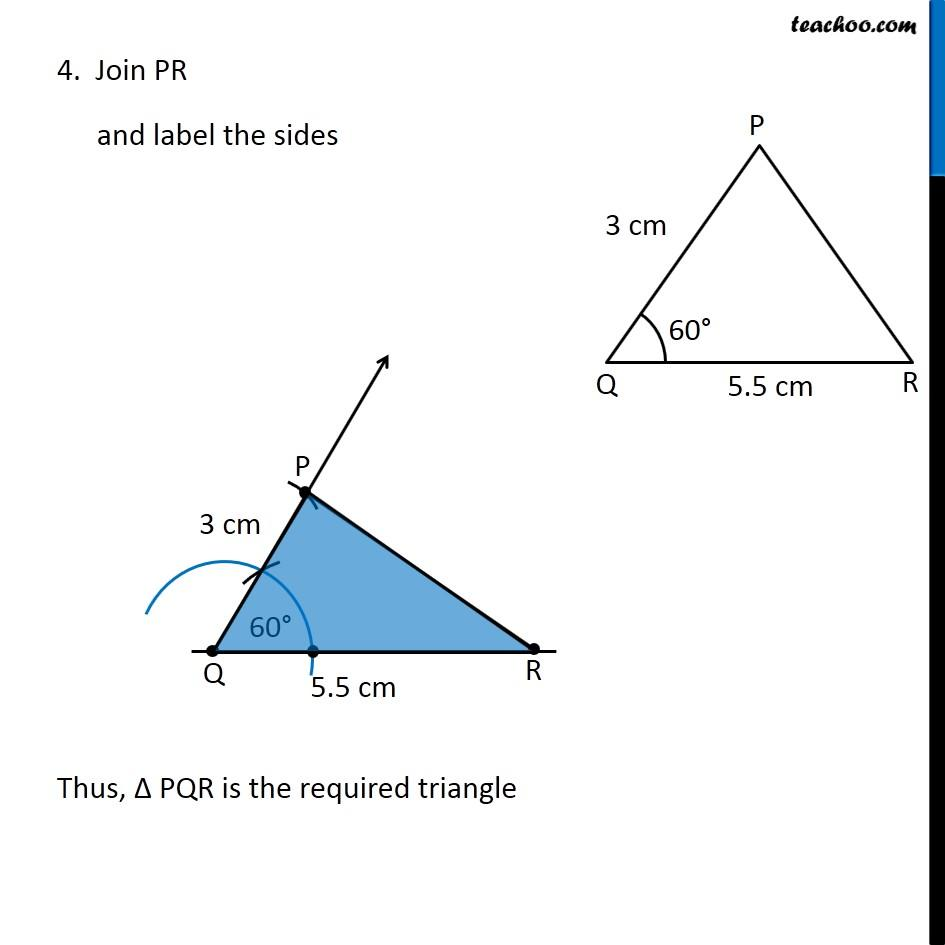Introducing your new favourite teacher - Teachoo Black, at only ₹83 per month

### Transcript

Example 2 Construct a triangle PQR, given that PQ = 3 cm, QR = 5.5 cm and ∠PQR = 60°. First we draw a rough sketch We follow these steps Steps of construction 1. Draw a line segment QR of length 5.5 cm 2. Now, we draw 60° from point Q Check Construction 11.3 of Chapter 11 Class 9 NCERT to see how to draw 60° 3. Taking Q as center, 3 cm as radius, we draw an arc Let the point where arc intersects the ray be point P 4. Join PR and label the sides Thus, Δ PQR is the required triangle